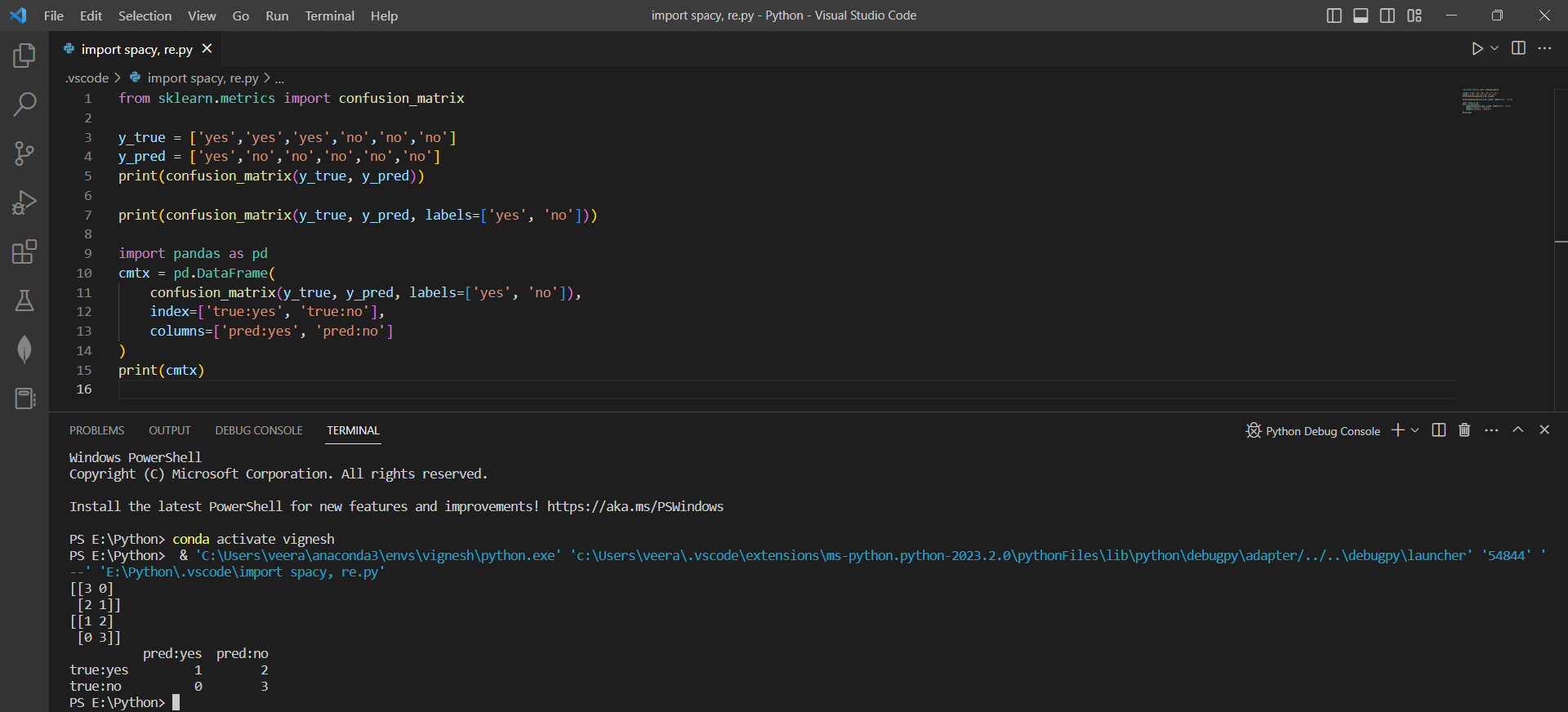# Computing and Visualizing a Confusion Matrix using Scikit-learn and Pandas in Pythonby vigneshchennai74Updated: Feb 27, 2023Solution Kit

In machine learning, classification models predict the class labels of unseen instances based on the available training data. The performance of these models needs to be evaluated to understand how well they perform on the data. A confusion matrix is one of the most common ways to evaluate classification model performance.

The confusion matrix is a table that outlines the classification results produced by a model. It contains information about the number of true positives, negatives, false positives, and negatives predicted by the model. These metrics are essential for evaluating model performance, and they can be used to calculate various other metrics such as precision, recall, and F1-score.

This code demonstrates how to compute and visualize a confusion matrix in Python using the sci-kit-learn and pandas libraries. The confusion_matrix function from sci-kit-learn calculates the confusion matrix for a set of true and predicted labels. The resulting matrix is then converted into a pandas DataFrame, which provides a more readable and interpretable representation of the matrix.

This code helps evaluate the performance of classification models by providing a quantitative measure of their performance on the given dataset. One can identify which classes are correctly classified and which are misclassified using a confusion matrix. We can use this information to adjust the model's parameters and improve performance. Additionally, the visualization of the confusion matrix can help communicate the model's performance to stakeholders clearly and concisely.

Here is an example of how to Computing and Visualizing a Confusion Matrix using Scikit-learn and Pandas in Python:Preview of the output that you will get on running this code from your IDE

### Code

In this solution we have used Scikit-Learn library.

1. Copy the code using the "Copy" button above, and paste it in a Python file in your IDE.
2. Run the file to create a simple confusion matrix

I hope you found this useful. I have added the link to dependent libraries, version information in the following sections.

I found this code snippet by searching for "Sci-kit learn how to print labels for confusion matrix" in kandi. You can try any such use case!

Note

If you want to print the confusion matrix with labels, you may try pandas and set the index and columns of the Dataframe by copying the next set of the code in our snippet.

### Environment Tested

I tested this solution in the following versions. Be mindful of changes when working with other versions.

1. The solution is created and tested on Vscode 1.75.1 version
2. The solution is created in Python 3.7.15 version
3. The solution is tested on the sci-kit-learn 1.0.2 version

Using this solution, we are able to create a simple confusion matrix using Scikit learn library in Python with simple steps. This process also facilities an easy-to-use, hassle-free method to create a hands-on working version of code which would help us get a confusion matrix in Python.

### Dependent Library

scikit-learnby scikit-learn

Python54584Version:1.2.2scikit-learn: machine learning in Python

Support
Quality
Security
Reuse

scikit-learnby scikit-learn

Python54584Version:1.2.2License: Permissive (BSD-3-Clause)

scikit-learn: machine learning in Python
Support
Quality
Security